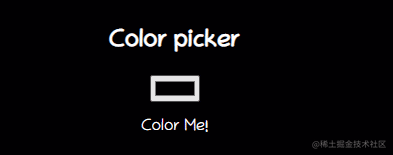你真的熟练运用 HTML5 了吗，这10 个酷炫的 H5 特性你会几个？「本文已参与好文召集令活动，点击查看：后端、大前端双赛道投稿，2万元奖池等你挑战！」

HTML5不是什么新鲜事。自初始版本（2008 年 1 月）以来，我们一直在使用它的几个功能。我再次仔细查看了 HTML5 功能列表。看看我发现了什么？到目前为止，我还没有真正使用过很多！

wanghao221.github.io/html-tips-t…

🍖 一、详情标签

<details>标签向用户提供按需详细信息。如果您需要按需向用户显示内容，请使用此标签。默认情况下，小部件是关闭的。打开时，它会展开并显示其中的内容。

<summary>标签用于<details>为它指定一个可见的标题。

<details>
<table>
<tr>
<th>#</th>
<th>Name</th>
<th>Location</th>
<th>Job</th>
</tr>
<tr>
<td>1</td>
<td>Huston</td>
<td>UI/UX</td>
</tr>
<tr>
<td>2</td>
<td>Bob</td>
<td>London</td>
<td>Machine Learning</td>
</tr>
<tr>
<td>3</td>
<td>Jack</td>
<td>Australia</td>
<td>UI Designer</td>
</tr>
<tr>
<td>4</td>
<td>Tapas</td>
<td>India</td>
<td>Blogger</td>
</tr>
</table>
</details>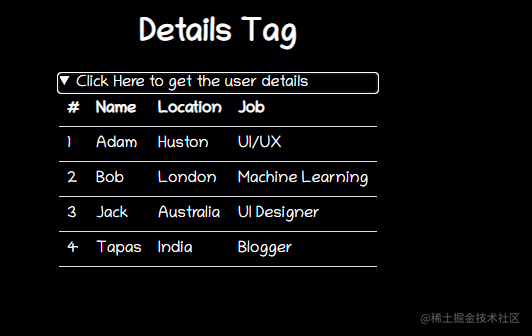🎶 二、内容可编辑

contenteditable是可以在元素上设置以使内容可编辑的属性。它适用于 DIV、P、UL 等元素。您必须指定它，例如，<element contenteditable="true|false">

==注意==: 当contenteditable元素上没有设置属性时，它将从其父元素继承。

<h2> Shoppping List(Content Editable) </h2>
<ul class="content-editable" contenteditable="true">
<li> 1. Milk </li>
<li> 3. Honey </li>
</ul>

==快速提示==

span 或 div 元素可以使用它进行编辑，您可以使用 CSS 样式向其中添加任何丰富的内容。这将比使用输入字段处理它要好得多。去试一试！

✨ 三、地图

<map>标签有助于定义图像映射。图像映射是其中包含一个或多个可点击区域的图像。地图标签带有一个<area>标签来确定可点击区域。可点击区域可以是这些形状、矩形、圆形或多边形区域之一。如果您不指定任何形状，它会考虑整个图像。

<div>
<img src="circus.jpg" width="500" height="500" alt="Circus" usemap="#circusmap">

<map name="circusmap">
<area shape="rect" coords="67,114,207,254" href="elephant.htm">
<area shape="rect" coords="222,141,318, 256" href="lion.htm">
<area shape="rect" coords="343,111,455, 267" href="horse.htm">
<area shape="rect" coords="35,328,143,500" href="clown.htm">
<area shape="circle" coords="426,409,100" href="clown.htm">
</map>
</div>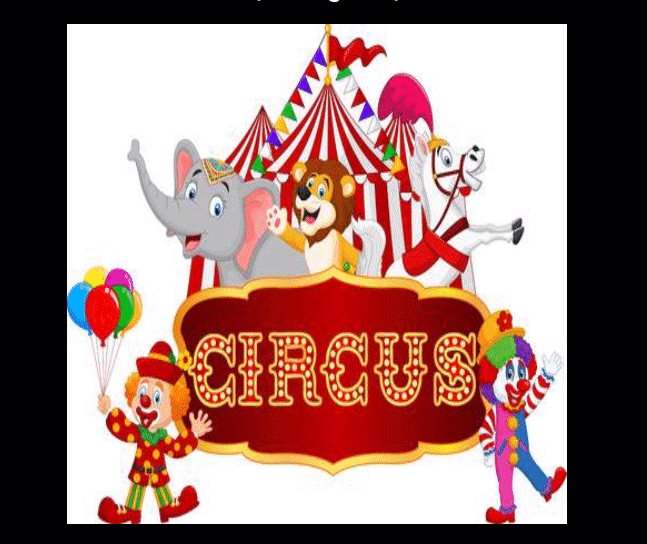==快速提示==

🏀 四、标记内容

<p> 你知道吗，你可以仅使用 HTML 标签 <mark>"突出显示有趣的东西"</mark></p>

==快速提示==

mark {
background-color: green;
color: #FFFFFF;
}

🎥 五、data-* 属性

data-* 属性由两部分组成：

• 属性名称不应包含任何大写字母，并且必须在前缀“data-”之后至少长一个字符
• 属性值可以是任何字符串

<h2> Know data attribute </h2>
<div
class="data-attribute"
id="data-attr"
data-custom-attr="You are just Awesome!">
I have a hidden secret!
</div>

<button onclick="reveal()">Reveal</button>

function reveal() {
document.getElementById('msg').innerHTML = `<mark>\${value}</mark>`;
}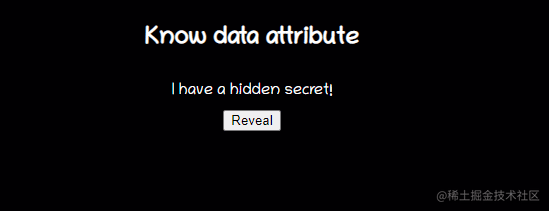==快速提示==

🏆 六、输出标签

<output>标签表示的运算的结果。通常，此元素定义将用于显示某些计算的文本输出的区域。

<form oninput="x.value=parseInt(a.value) * parseInt(b.value)">
<input type="number" id="a" value="0">
* <input type="number" id="b" value="0">
= <output name="x" for="a b"></output>
</form>

==快速提示==

🎻 七、数据列表

<datalist>标签指定了一个预定义选项列表，并允许用户向其中添加更多选项。它提供了一项autocomplete功能，允许您通过预先输入获得所需的选项。

<form action="" method="get">
<label for="fruit">Choose your fruit from the list:</label>
<input list="fruits" name="fruit" id="fruit">
<datalist id="fruits">
<option value="Apple">
<option value="Orange">
<option value="Banana">
<option value="Mango">
</datalist>
<input type="submit">
</form>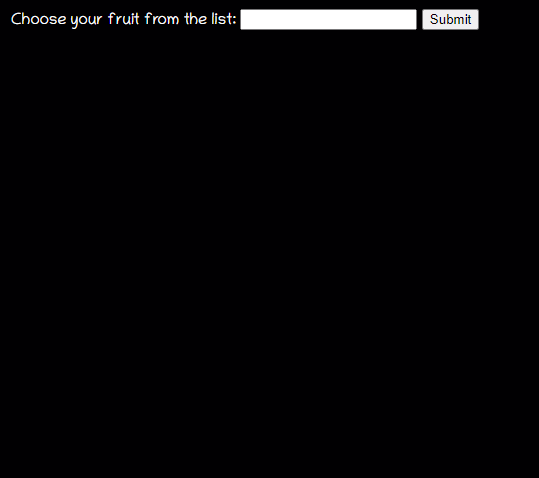==快速提示==

🧿 八、范围（滑块）

range是给定滑块类型范围选择器的输入类型。

<form method="post">
<input
type="range"
name="range"
min="0"
max="100"
step="1"
value=""
onchange="changeValue(event)"/>
</form>
<div class="range">
<output id="output" name="result">  </output>
</div>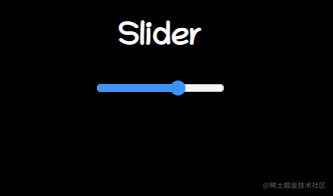==快速提示==

HTML5 中没有叫slider的！

⏰ 九、Meter

<label for="home">/home/atapas</label>
<meter id="home" value="4" min="0" max="10">2 out of 10</meter><br>

<label for="root">/root</label>
<meter id="root" value="0.6">60%</meter><br>

==快速提示==

<progress id="file" value="32" max="100"> 32% </progress>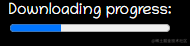💌 十、Inputs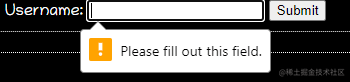placeholder="6-20 chars, at least 1 digit, 1 uppercase and one lowercase letter"
pattern="^(?=.*\d)(?=.*[a-z])(?=.*[A-Z]).{6,20}\$" autofocus required>

<input type="color" onchange="showColor(event)">
<p id="colorMe">Color Me!</p>